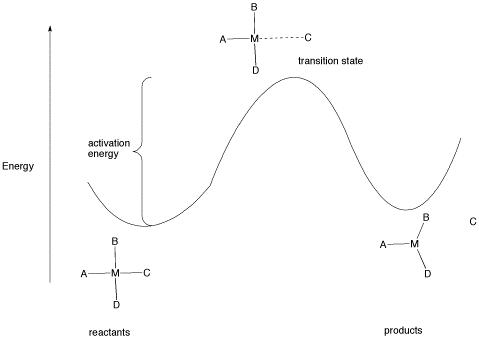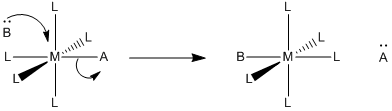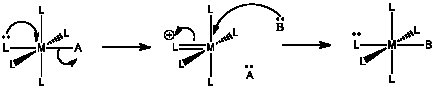# LS5. Activation Parameters

$$\newcommand{\vecs}{\overset { \rightharpoonup} {\mathbf{#1}} }$$ $$\newcommand{\vecd}{\overset{-\!-\!\rightharpoonup}{\vphantom{a}\smash {#1}}}$$$$\newcommand{\id}{\mathrm{id}}$$ $$\newcommand{\Span}{\mathrm{span}}$$ $$\newcommand{\kernel}{\mathrm{null}\,}$$ $$\newcommand{\range}{\mathrm{range}\,}$$ $$\newcommand{\RealPart}{\mathrm{Re}}$$ $$\newcommand{\ImaginaryPart}{\mathrm{Im}}$$ $$\newcommand{\Argument}{\mathrm{Arg}}$$ $$\newcommand{\norm}{\| #1 \|}$$ $$\newcommand{\inner}{\langle #1, #2 \rangle}$$ $$\newcommand{\Span}{\mathrm{span}}$$ $$\newcommand{\id}{\mathrm{id}}$$ $$\newcommand{\Span}{\mathrm{span}}$$ $$\newcommand{\kernel}{\mathrm{null}\,}$$ $$\newcommand{\range}{\mathrm{range}\,}$$ $$\newcommand{\RealPart}{\mathrm{Re}}$$ $$\newcommand{\ImaginaryPart}{\mathrm{Im}}$$ $$\newcommand{\Argument}{\mathrm{Arg}}$$ $$\newcommand{\norm}{\| #1 \|}$$ $$\newcommand{\inner}{\langle #1, #2 \rangle}$$ $$\newcommand{\Span}{\mathrm{span}}$$$$\newcommand{\AA}{\unicode[.8,0]{x212B}}$$

### LS5. Activation Parameters

The rate law shows how the rate of a reaction depends on concentrations of different species in solution. The proportionality constant, k, is called the rate constant. It contains other information about the energetic requirements of the reaction.

All reactions must overcome activation barriers in order to occur. The activation barrier is the sum of the energy that must be expended to get the reaction going. An activation barrier is often thought of, cartoonishly, as a hill the molecule has to climb over during the reaction. Once, there, it can just slide down the other side of the hill to become products. At the top of the hill, the molecule exists in what is called the "transition state". At the transition state, the structure is somewhere between its original form and the structure of the products.Figure LS5.1. The activation barrier for a ligand dissociation step.

The type of diagram shown in figure LS6.1 is sometimes called a "reaction progress diagram". It shows energy changes in the system as a reaction proceeds. One or more activation barriers may occur along the reaction pathways, as various elementary steps occur in the reaction. In the above case, it is easy to imagine the source of the energy barrier, because some energy must be expended to break the bond to ligand C.

However, after that barrier is passed, energy is lowered again. This can happen for several reasons. Once C has separated from the metal complex, it is free to vibrate, tumble, roll and zip around all on its own. That means it can put its energy into any of those modes, independently of the metal complex. As a result, the entropy of the system increases. That lowers the overall "free energy" of the system. In addition, there may be some relief of crowding as the molecule changes from a four-coordinate complex to a three-coordinate complex, so strain energy is also lowered.

#### Problem LS5.1.

Make drawings depicting the relationship between reaction progress and energy for the following cases:

1. a new ligand binds to a four-coordinate complex, forming a five coordinate complex.
2. a two-step process in which a new ligand binds to a four-coordinate complex, forming a five coordinate complex, and then an old ligand dissociates to form a new, four-coordinate complex.

The rate constant gives direct insight into what is happening at the transition state, because it gives us the energy difference between the reactants and the transition state. Based on that information, we get some ideas of what is happening on the way to the transition state.

The rate constant can be broken down into pieces. Mathematically, it is often expressed as

$k = \left ( RT \over Nh \right ) e^{- \Delta G \ddagger \over RT}$

In which R = the ideal gas constant, T = temperature, N = Avogadro's number, h = Planck's constant and Δ G = the free energy of activation.

The ideal gas constant, Planck's constant and Avogadro's number are all typical constants used in modeling the behaviour of molecules or large groups of molecules. The free energy of activation is essentially the energy requirement to get a molecule (or a mole of them) to undergo the reaction.

Note that k depends on just two variables:

• Δ G or the energy required for the reaction
• T or the temperature of the surroundings, which is an index of the available energy

The ratio of activation free energy to temperature compares the energy needs to the energy available. The more energy available compared to the energy needed, the lower this ratio becomes. As a result, the exponential part of the function becomes larger (since the power has a minus sign). That makes the rate constant bigger, and the reaction becomes faster.

The activation free energy is constant for a given reaction. It can be broken down in turn to:

$\Delta G^{\ddagger} = \Delta H^{\ddagger} - T \Delta S^{\ddagger}$

in which Δ H = activation enthalpy and Δ S = activation entropy.

The activation enthalpy is the energy required for the reaction. The activation entropy deals with how the energy within the molecule must be redistributed for the reaction to occur. These two parameters can be useful in understanding events leading to the transition state.

For example, in ligand substitution, an associative pathway is marked by low enthalpy of activation but a negative entropy of activation. The low enthalpy of activation results because bonds don't need to be broken before the transition state, so it doesn't cost much to get there. That's favourable and makes the reaction easier. However, a decrease in entropy means that energy must be partitioned into fewer states. That's not favourable and makes the reaction harder. The reason the energy must be redistributed this way is that two molecules (the metal complex and the new ligand) are coming together to make one bigger molecule. They can no longer move independently of each other, and all of their combined energy must be reapportioned together, with a more limited range of vibrational, rotational and translational states to use for that purpose.

• Associative pathway: more bond making than bond breaking; lower enthalpy needs
• Associative pathway: two molecules must be aligned and come together; fewer degrees of freedom for energy distribution; decrease in entropy

On the other hand, the dissociative pathway is marked by a higher enthalpy of activation but a positive entropy of activation. The higher enthalpy of activation results because a bond must be broken in the rate determining step. That's not favourable. However, the molecule breaks into two molecules in the rate determining step. these two molecules have more degrees of freedom in which to partition their energy than they did as one molecule. That's favourable.

• Dissociative pathway: more bond breaking in rate determining step, higher enthalpy needs
• Dissociative pathway: one molecule converts to two molecules in rate determining step, greater degrees of freedom in two independently moving molecules, entropy increases

Thus, looking at the activation parameters can reveal a lot about what is going on in the transition state.

#### Problem LS5.2.

What factor(s) other than entropy might raise the free energy of the transition state going into an associative step between a metal complex and an incoming ligand? (What factor might make the first, associative step slower than the second, dissociative step?)

#### Problem LS5.3.

Other mechanisms for ligand substitution are also possible. The following case is referred to as an associative interchange (IA).a) Describe in words what happens in an associative interchange.

b) Predict the rate law for the reaction.

c) Qualitatively predict the activation entropy and enthalpy, compared with

i) an associative mechanism and

ii) a dissociative mechanism.

#### Problem LS5.4.

For the following mechanism:a) Describe in words what is happening.

b) Predict the rate determining step.

c) Predict the rate law for the reaction.

d) Qualitatively predict the activation entropy and enthalpy, compared with

i) both an associative mechanism and

ii) a dissociative mechanism.

e) Suggest some ligands that may be able to make this mechanism occur.

## Contributors

This page titled LS5. Activation Parameters is shared under a not declared license and was authored, remixed, and/or curated by Chris Schaller.